# Neural Networks in R

RDeep Learning

This post explores how to create a simple neural network to learn a linear function and a non-linear function using both standard R and the Torch library for R.

Author

Jonny Law

Published

February 2, 2021

w$grad } network_gradient_torch(x = torch_tensor(as.matrix(x_test)), y = torch_tensor(as.matrix(y_test)), w = torch_tensor(as.matrix(w), requires_grad = TRUE)) torch_tensor 0.1510 0.0542 [ CPUFloatType{2,1} ] We can fit this simple model by writing a training loop which updates the parameters using gradient descent. We keep track of the loss function at each iteration of gradient descent and plot it. observed <- tibble(x, y) %>% sample_n(50) training_loop <- function(x, y, init_w, epochs, learning_rate) { # Record all changes to parameters and training loss ws <- matrix(NA_real_, nrow = epochs + 1, ncol = length(init_w)) ws[1,] = init_w losses <- numeric(length(y)) for (i in seq_len(epochs)) { # Pad the input with 1 for intercept/bias input <- cbind(rep(1, times = length(x)), x) # Made a prediction using the model pred <- layer(input, ws[i,], function(z) z) # We can calculate and log/print the loss losses[i] <- loss(y, pred) # calculate the gradient at this point gradient <- network_gradient(x = input, y = y, w = ws[i, ]) # Update using gradient descent ws[i + 1, ] <- gradient_step(learning_rate, ws[i, ], gradient) } list(weights = ws, losses = losses) } out <- training_loop(x = scale(observed$x), y = scale(observed$y), c(1, 1), 500, 1e-4) qplot(x = seq_along(out$losses), y = out$losses, geom = "line") + labs(x = "Epoch", y = "Loss")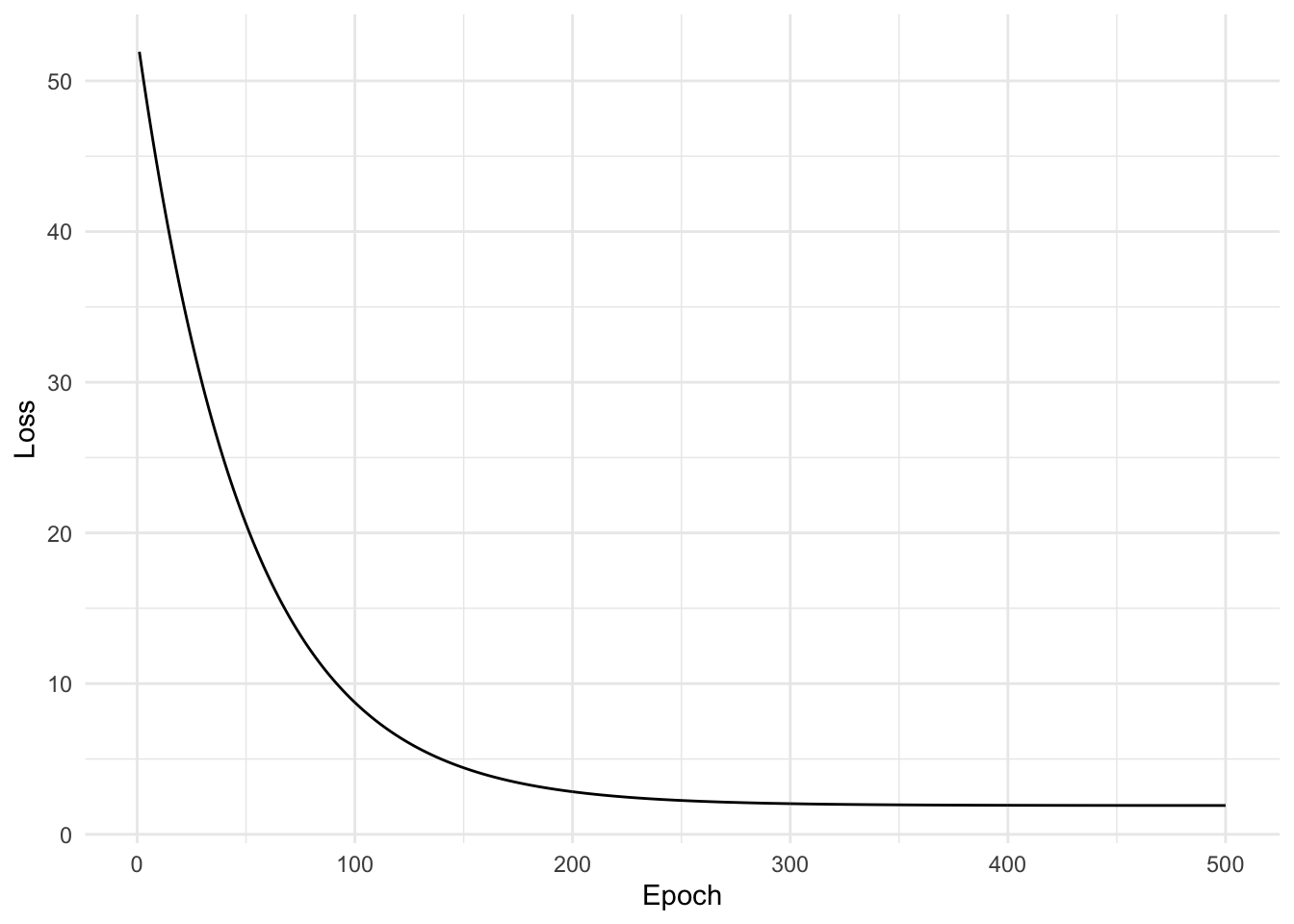Since this model is so small, consisting of only two weights. We can plot the actual function learned by the model using geom_abline. ggplot(observed, aes(scale(x), scale(y))) + geom_point() + geom_abline(intercept = out$weights[501, 1], slope = out$weights[501,2]) + labs(x = "x", y = "y")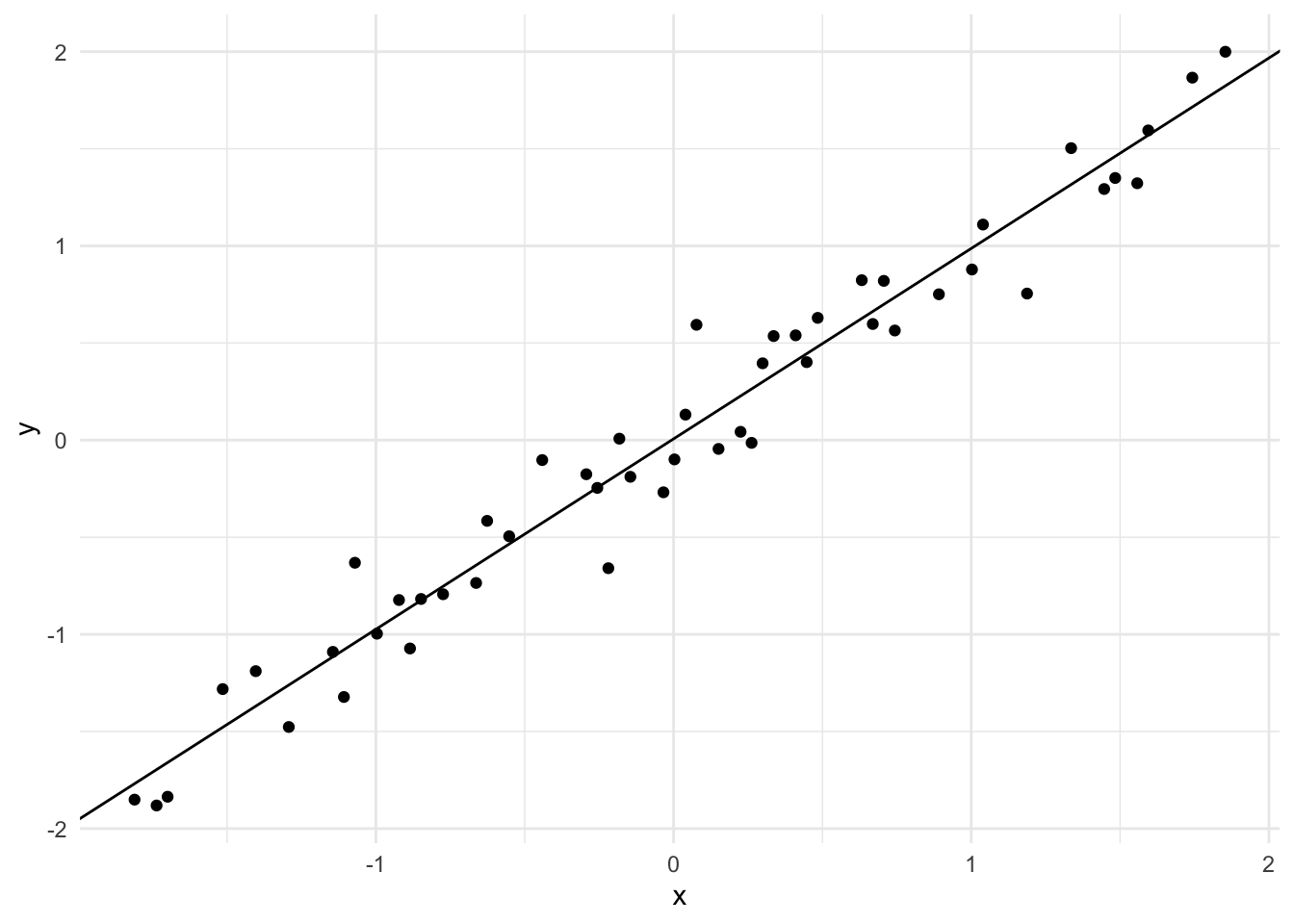We can try to use this model on a simple non-linear regression problem, of course we probably won’t do very well here! We define the regression problem as $y \sim \mathcal{N}(4\sin(x), 1^2).$ We plot the true function and the observed values in red below. n <- 100 x <- seq(-5, 5, length.out=n) y <- 4 * sin(x) y_obs <- 4 * sin(x) + rnorm(n, sd = 1) non_linear <- tibble(x, y_obs) %>% sample_n(20) qplot(x, y, geom = "line") + geom_point(data = non_linear, aes(x = x, y = y_obs, colour = "Observed")) + theme(legend.position = "none")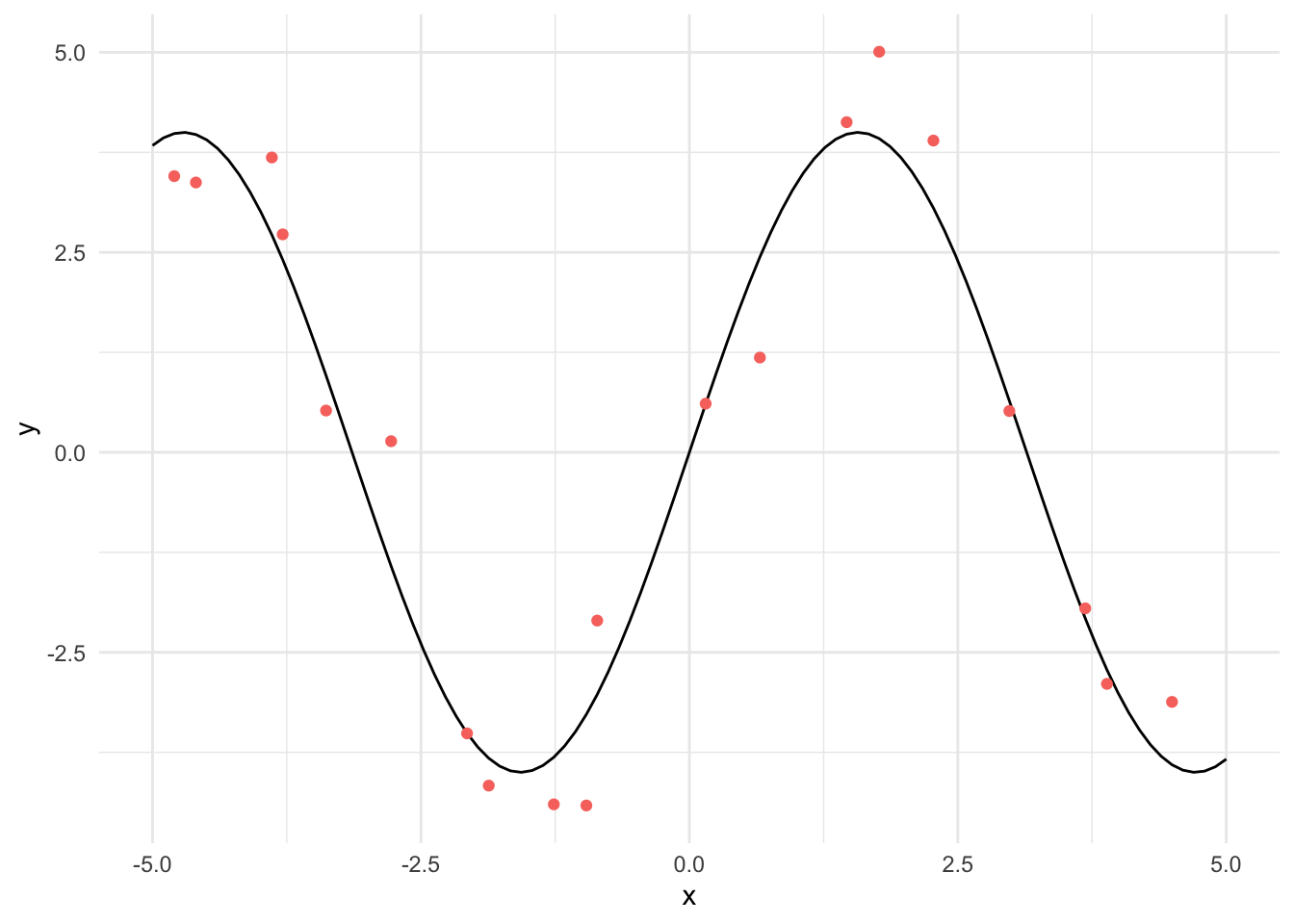out <- training_loop(non_linear$x, non_linear$y_obs, c(1, 1), 200, 1e-4) qplot(x = seq_along(out$losses), y = out$losses, geom = "line") + labs(x = "Epoch", y = "Loss")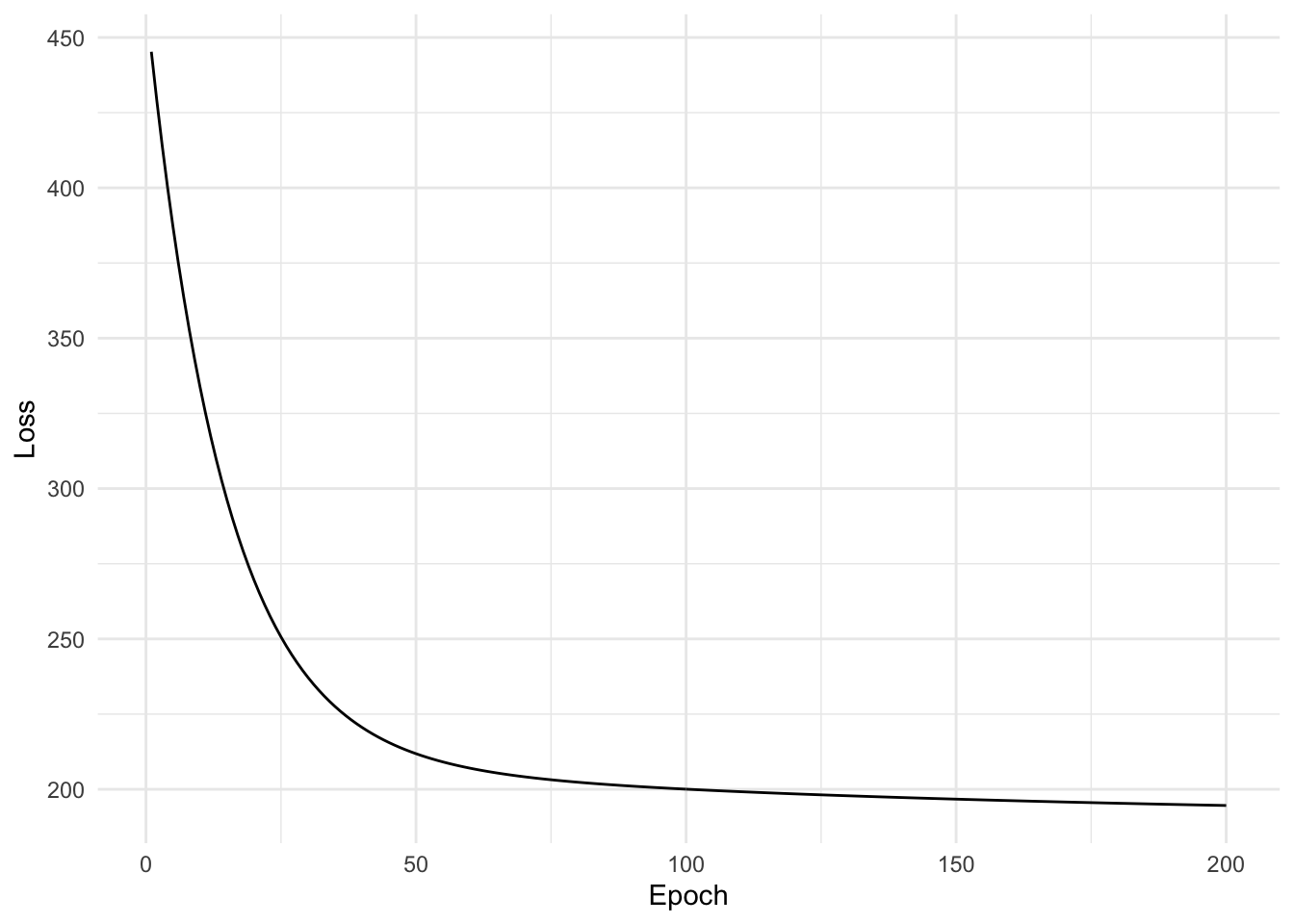We can then plot the learned function against the observed values and the true function. We can see that a straight line is not a good fit for this data, we need more flexibility in the network. qplot(x, y, geom = "line", colour = "truth") + geom_point(data = non_linear, aes(x, y_obs, colour = "observed")) + geom_abline(intercept = out$weights[201,1], slope = out$weights[201,1]) + theme(legend.position = "none")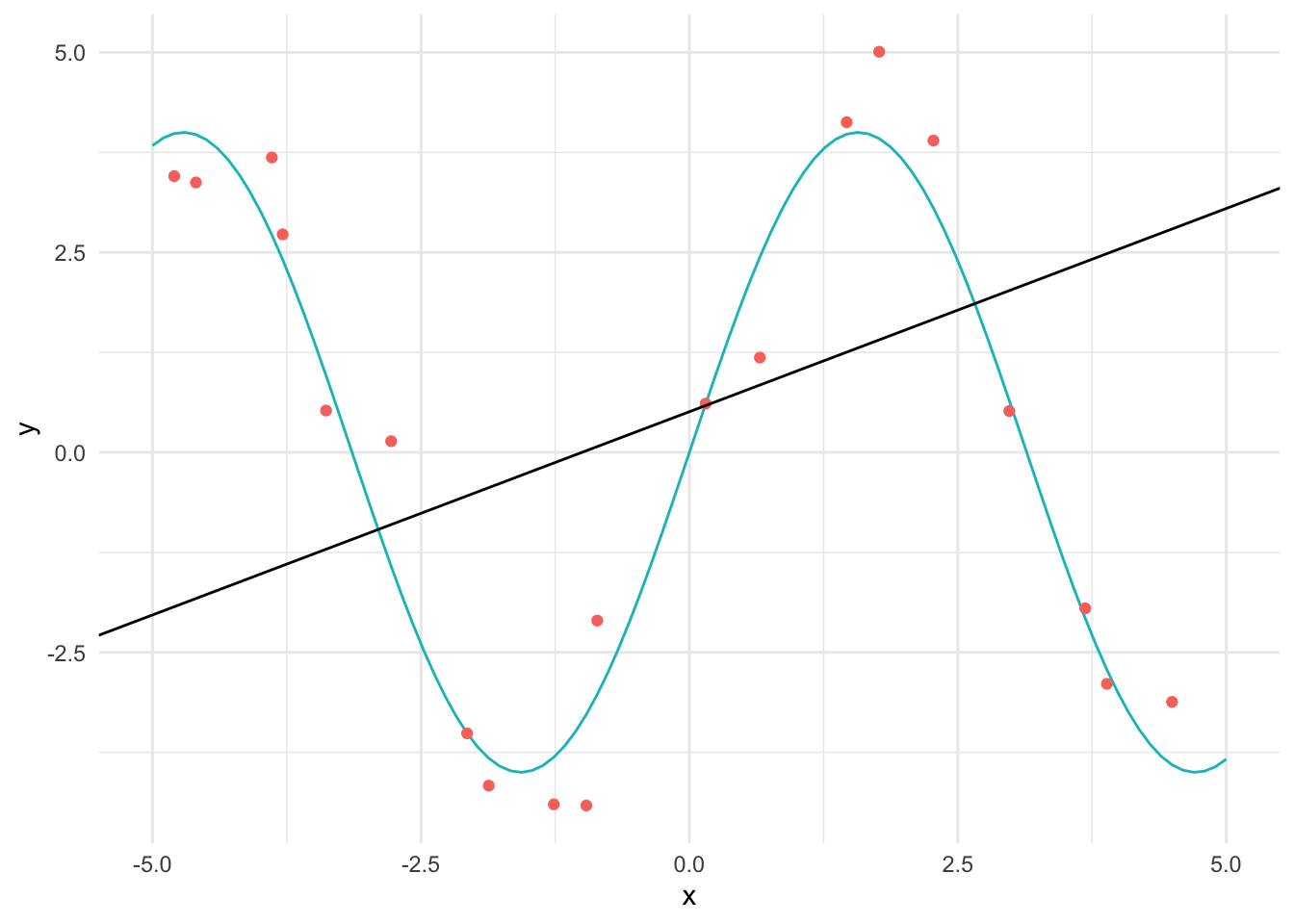## Using Torch If we want to approximate a non-linear function, we best use non-linear activation functions. We can calculate the derivative of each layer using automatic differentiation. We will use the R Torch library. We now initialise a torch tensor with the same values as x and pass it through the layers. We must re-write the layer and loss functions assuming the input is a torch_tensor. First we will re-write the linear example using Torch model <- nn_linear(1, 1) loss <- function(y, pred) { (y - pred)$pow(2)$sum() } train_torch <- function(x, y, model, loss, epochs) { alpha <- 1e-4 x_in <- torch_tensor(as.matrix(x)) for (i in seq_len(epochs)) { pred <- model(x_in) losses <- loss(torch_tensor(y), pred) model$zero_grad()
losses$backward() if (i %% 10 == 0) cat("Epoch: ", i, " Loss: ", losses$item(), "\n")
model$parameters %>% purrr::walk(function(param) param$sub_(alpha * param$grad)) }) } model } trained_model <- train_torch(scale(observed$x), scale(observed$y), model, loss, 200) Epoch: 10 Loss: 58.6479 Epoch: 20 Loss: 48.48629 Epoch: 30 Loss: 40.14468 Epoch: 40 Loss: 33.2971 Epoch: 50 Loss: 27.67593 Epoch: 60 Loss: 23.06152 Epoch: 70 Loss: 19.27356 Epoch: 80 Loss: 16.16403 Epoch: 90 Loss: 13.6114 Epoch: 100 Loss: 11.51595 Epoch: 110 Loss: 9.795779 Epoch: 120 Loss: 8.383685 Epoch: 130 Loss: 7.224489 Epoch: 140 Loss: 6.272895 Epoch: 150 Loss: 5.491727 Epoch: 160 Loss: 4.850457 Epoch: 170 Loss: 4.324034 Epoch: 180 Loss: 3.891886 Epoch: 190 Loss: 3.53713 Epoch: 200 Loss: 3.245904  ggplot(observed, aes(scale(x), scale(y))) + geom_point() + geom_abline(intercept = trained_model$parameters$bias$item(), slope = trained_model$parameters$weight$item())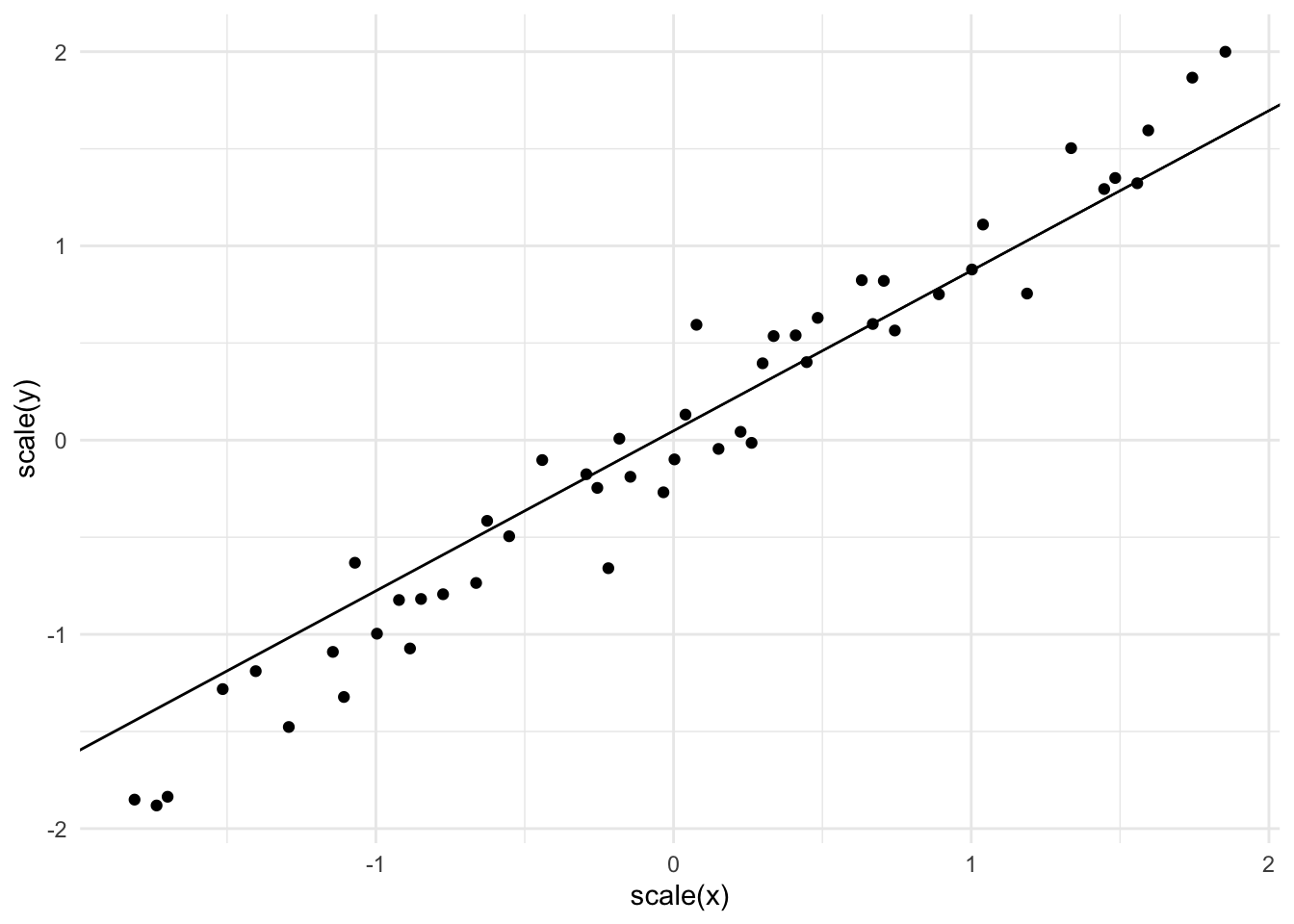Try the non-linear example with multiple layers and a non-linear activation function from the first layer (where the input goes). We’ll also try a different optimizer, Adam. train_torch <- function(x, y, model, loss, epochs, learning_rate) { x_in <- x optimiser <- optim_adam(model$parameters, lr = learning_rate)
for (i in seq_len(epochs)) {
pred <- model(x_in)
losses <- loss(y, pred)
model$zero_grad() losses$backward()
if (i %% 10 == 0)
cat("Epoch: ", i, "   Loss: ", losses$item(), "\n") optimiser$step()
}
model
}

model <- nn_sequential(
nn_linear(1, 64),
nn_relu(),
nn_linear(64, 1)
)

trained_model <-
train_torch(torch_tensor(as.matrix(non_linear$x)), torch_tensor(as.matrix(non_linear$y_obs)),
model,
nnf_mse_loss,
100,
0.1)
Epoch:  10    Loss:  6.853224
Epoch:  20    Loss:  2.670638
Epoch:  30    Loss:  1.007208
Epoch:  40    Loss:  0.7993301
Epoch:  50    Loss:  0.683284
Epoch:  60    Loss:  0.6011851
Epoch:  70    Loss:  0.5383755
Epoch:  80    Loss:  0.5088955
Epoch:  90    Loss:  1.185604
Epoch:  100    Loss:  0.5675938 

We can plot the predictions alongside the observed values and the true function.

predictions <- tibble(x, prediction = as_array(trained_model(torch_tensor(x)\$unsqueeze(-1))))

qplot(x, y, geom = "line", colour = "truth") +
geom_line(data = predictions, aes(x, prediction, colour = "fitted")) +
geom_point(data = non_linear, aes(x, y_obs, colour = "observed")) +
labs(colour = "") +
theme(legend.position = c(0.9, 0.9))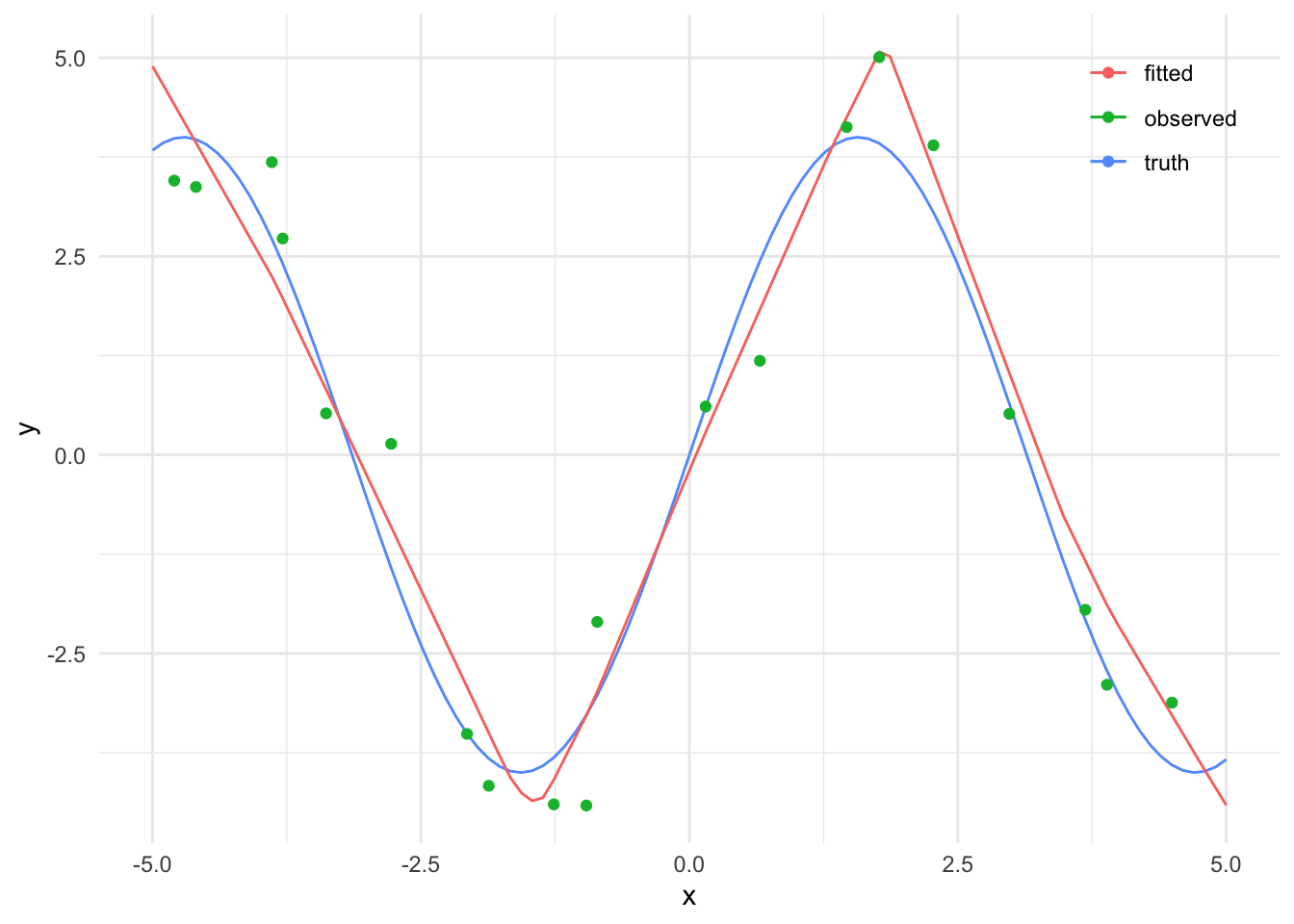## Citation

BibTeX citation:
@online{law2021,
author = {Jonny Law},
title = {Neural {Networks} in {R}},
date = {2021-02-02},
langid = {en}
}